# Poincaré-Bertrand formula

(diff) ← Older revision | Latest revision (diff) | Newer revision → (diff)

A formula for rearranging the order of integration in iterated improper integrals of Cauchy principal value type (cf. Improper integral).

Letbe a simple closed or open smooth curve in the complex plane, let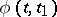be a function defined on(generally speaking complex-valued) and satisfying a uniform Hölder condition with respect toand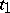, and letbe a fixed point onwhich is not an end-point ifis open. Then one has the Poincaré–Bertrand formula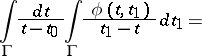(1)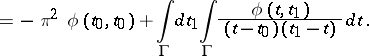The formula is true under more general assumptions on the curveand the function(see ). If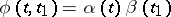, where,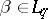,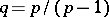, equation (1) is true for almost-all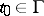(see , ). If the curveis closed and the functiondepends on one variable only, then equation (1) takes the form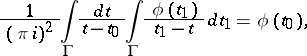(2)

and holds for all or almost-all, depending (respectively) on whethersatisfies a Hölder condition or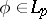,. Equation (2) is also called the Poincaré–Bertrand formula.

Analogues of formula (1) have been constructed for multiple integrals (see ).

Formula (1) was obtained, under certain conditions, by G.H. Hardy (see ) before H. Poincaré (see ) and G. Bertrand (see , ).

How to Cite This Entry:
Poincaré-Bertrand formula. Encyclopedia of Mathematics. URL: http://encyclopediaofmath.org/index.php?title=Poincar%C3%A9-Bertrand_formula&oldid=14405
This article was adapted from an original article by B.V. Khvedelidze (originator), which appeared in Encyclopedia of Mathematics - ISBN 1402006098. See original article